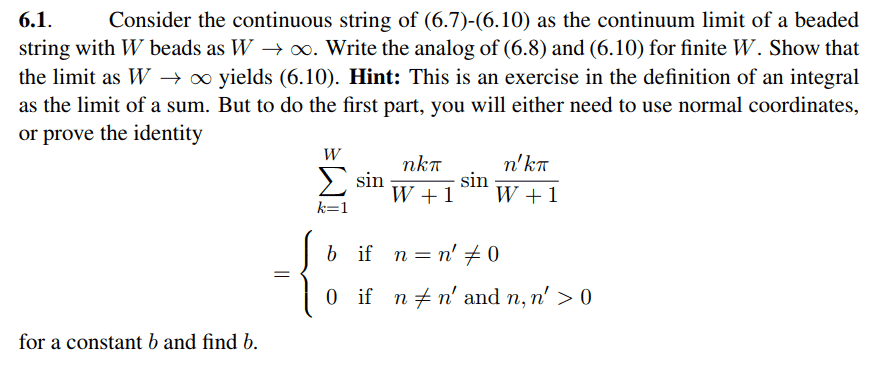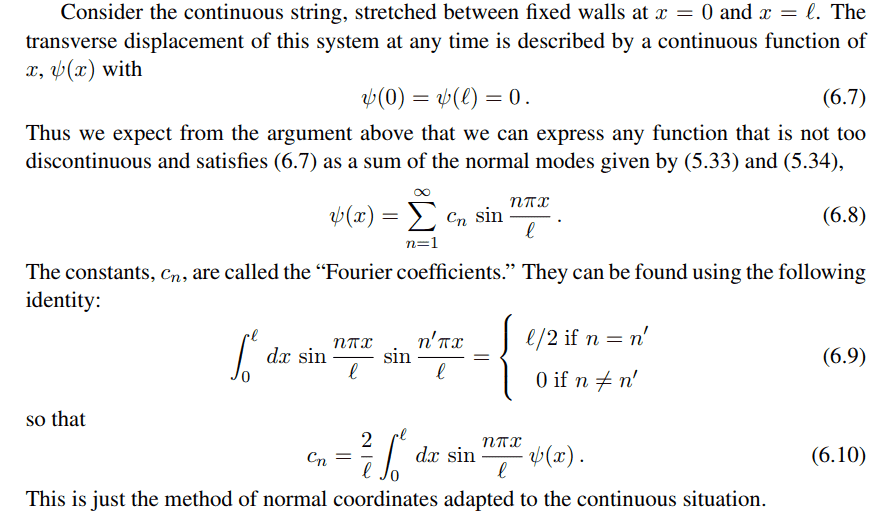# The sum of this series of the product of 2 sine functions

Miles123K

## Homework Statement

I have encountered this problem from the book The Physics of Waves and in the end of chapter six, it asks me to prove the following identity as part of the operation to prove that as the limit of ##W## tends to infinity, the series becomes an integral. The series involved is as follows:

##\sum_{n=1}^{W} sin(\frac {nk\pi} {W+1}) sin(\frac {n'k\pi} {W+1})##
I need to prove that:
##\sum_{n=1}^{W} sin(\frac {nk\pi} {W+1}) sin(\frac {n'k\pi} {W+1})=b## when ##n=n'## and ##=0## when ##n \neq n'## where ##n, n'## are positive integers.

## Homework Equations

Trigonometric Identities?

## The Attempt at a Solution

I used the identities to convert the series into the following:
##\sum_{n=1}^{W} \frac 1 2 (cos( \frac {(n-n')k \pi} {W+1}) - cos( \frac {(n+n')k \pi} {W+1}))##
By writing out the terms and regrouping, I concluded that:
##\sum_{n=1}^{W} cos( \frac {(n-n')k \pi} {W+1}) = \sum_{n=1}^{\frac 1 2 W} cos( \frac {(n-n')k \pi} {W+1}) + cos( \frac {(n-n') (W+1-k) \pi} {W+1})## for even ##W##
and:
##\sum_{n=1}^{W} cos( \frac {(n-n')k \pi} {W+1}) = \sum_{n=1}^{\frac {2W+1} {2}} cos( \frac {(n-n')k \pi} {W+1}) + cos( \frac {(n-n') (W+1-k) \pi} {W+1})## for odd ##W##.
In both cases,
##\sum_{n=1}^{W} cos( \frac {(n-n')k \pi} {W+1}) = 2 cos( \frac {\pi} {2} (n-n')) cos( \frac {(n-n') (W+1-2k) \pi} {2(W+1)})## which will be zero for all odd ##(n-n')##.
The same procedure goes for
##\sum_{n=1}^{W} (cos( \frac {(n+n')k \pi} {W+1})##, whereas the series become ##2 cos( \frac {\pi} {2} (n+n')) cos( \frac {(n+n') (W+1-2k) \pi} {2(W+1)})## which will be zero for all odd ##(n+n')## since if ##(n-n')## is odd, ##(n+n')## is also odd. Here I appear to have proved the identity that the series is zero for all ##(n-n')## is odd; however, I could not find any method to prove it for cases when ##(n-n')## is even. I really need help with this. Can someone point me at a certain direction?

## Answers and Replies

Homework Helper
There are subtle differences between your problem statement and what I found here , also at the end of chapter 6:and, for completeness:and yes, trigonometric identities is the way to go.

#### Attachments

Miles123K
There are subtle differences between your problem statement and what I found here , also at the end of chapter 6:
View attachment 239930

and, for completeness:

View attachment 239931

and yes, trigonometric identities is the way to go.
Ahh yes thank you for your addition. However, what should my next step be?

Homework Helper
It's not really an addition...For ##\ n=n'\ ## you are done quickly.
what should my next step be
sorting out which trig identities you needMiles123K
It's not really an addition...For ##\ n=n'\ ## you are done quickly.
sorting out which trig identities you need##\sum_{k=1}^{W} cos( \frac {(n-n')k \pi} {W+1}) = \sum 2 cos( \frac {\pi} {2} (n-n')) cos( \frac {(n-n') (W+1-2k) \pi} {2(W+1)})##
##\sum_{k=1}^{W} cos( \frac {(n+n')k \pi} {W+1}) =\sum 2 cos( \frac {\pi} {2} (n+n')) cos( \frac {(n+n') (W+1-2k) \pi} {2(W+1)})##
These two equations that I concluded could only prove the case where #n+n'# is even. I don't know how to prove the same for the case where ##n+n'## is even. I'll try to prove the case where ##n=n'## now. However, the question about even ##n+n'## is still unsolved.

Last edited:
Miles123K
I made a mistake above, the upper limit of the sum should be like this:
##\sum_{k=1}^{W} cos( \frac {(n-n')k \pi} {W+1}) = \sum_{k=1}^{\frac {W-1} {2}} cos( \frac {(n-n')k \pi} {W+1}) + cos( \frac {(n-n') (W+1-k) \pi} {W+1})## for cases where ##W## is odd. Also, for some reason I accidentally made all the limit in terms of ##n##, they should be in terms of ##k##

Staff Emeritus
Homework Helper
•Miles123K
Miles123K
At this point, I'd express the cosines as the real part of a complex exponential and then sum the resulting geometric series. If you'd rather just use trig identities, you'll find them here:

https://en.wikipedia.org/wiki/List_of_trigonometric_identities#Other_sums_of_trigonometric_functions

Thanks! I took the approach of complex exponential and was able to complete the proof. Could you check it?

##\sum_{k=1}^{W} cos( \frac {(n-n')k \pi} {W+1}) = \sum_{k=1}^{W} (e^{i \frac {(n-n') \pi} {W+1}})^{k} ##
The summation of the above series would be
##\frac {e^{\frac {(n-n')\pi} {W+1}} - e^{(n-n')\pi}} {1 - e^{\frac {(n-n')\pi} {W+1}}}##
since ##e^{(n-n')\pi} = 1## for even ##n-n'##,
##\sum_{k=1}^{W} cos( \frac {(n-n')k \pi} {W+1}) = -1##
The same goes for ##\sum_{k=1}^{W} cos( \frac {(n+n')k \pi} {W+1}) = -1##
##\sum_{k=1}^{W} \frac 1 2 (\cos( \frac {(n-n')k \pi} {W+1}) - \cos( \frac {(n+n')k \pi} {W+1}))## therefore becomes zero. This way the cases where ##n-n'## is even are also proved.

For ##n=n'##, the expression becomes
##\sum_{n=1}^{W} sin^2(\frac {nk\pi} {W+1})##, which is equivalent to
##\sum_{n=1}^{W} \frac 1 2 - \frac 1 2 cos(\frac {2nk\pi} {W+1})##
Going through the same process of summation, I get
##\frac {e^{\frac {2n\pi} {W+1}} - e^{2n\pi}} {1 - e^{\frac {2n\pi} {W+1}}}##
since ##e^{2n\pi} = 1## for all integer ##n##, the above expression becomes
##\frac {e^{\frac {2n\pi} {W+1}} - 1} {1 - e^{\frac {2n\pi} {W+1}}} = -1##
and thus ##\sum_{n=1}^{W} \frac 1 2 - \frac 1 2 cos(\frac {2nk\pi} {W+1})## becomes
##\sum_{n=1}^{W} \frac 1 2 + \frac 1 2 = W##
Combining with the context of the question, ##b = W##

Is this the correct conclusion?

Staff Emeritus
Homework Helper
##\sum_{k=1}^{W} \cos( \frac {(n-n')k \pi} {W+1}) = \sum_{k=1}^{W} (e^{i \frac {(n-n') \pi} {W+1}})^{k} ##
Only the real part of the series on the right is equal to the series on the left.
The summation of the above series would be
##\frac {e^{\frac {(n-n')\pi} {W+1}} - e^{(n-n')\pi}} {1 - e^{\frac {(n-n')\pi} {W+1}}}##
since ##e^{(n-n')\pi} = 1## for even ##n-n'##,
##\sum_{k=1}^{W} cos( \frac {(n-n')k \pi} {W+1}) = -1##
The same goes for ##\sum_{k=1}^{W} cos( \frac {(n+n')k \pi} {W+1}) = -1##
##\sum_{k=1}^{W} \frac 1 2 (\cos( \frac {(n-n')k \pi} {W+1}) - \cos( \frac {(n+n')k \pi} {W+1}))## therefore becomes zero. This way the cases where ##n-n'## is even are also proved.
You're missing a factor of ##i## in the exponents, but it otherwise looks fine. You might find it interesting to see if you can use this technique to solve the ##n-n'## being odd case. Note that you can start the summation from ##k=0##, which simplifies this case a little bit.

For ##n=n'##, the expression becomes
##\sum_{n=1}^{W} sin^2(\frac {nk\pi} {W+1})##, which is equivalent to
##\sum_{n=1}^{W} \frac 1 2 - \frac 1 2 cos(\frac {2nk\pi} {W+1})##
Going through the same process of summation, I get
##\frac {e^{\frac {2n\pi} {W+1}} - e^{2n\pi}} {1 - e^{\frac {2n\pi} {W+1}}}##
since ##e^{2n\pi} = 1## for all integer ##n##, the above expression becomes
##\frac {e^{\frac {2n\pi} {W+1}} - 1} {1 - e^{\frac {2n\pi} {W+1}}} = -1##
and thus ##\sum_{n=1}^{W} \frac 1 2 - \frac 1 2 cos(\frac {2nk\pi} {W+1})## becomes
##\sum_{n=1}^{W} \frac 1 2 + \frac 1 2 = W##
Combining with the context of the question, ##b = W##

Is this the correct conclusion?
I used Mathematica to calculate the actual sum for few values of ##n##, and it said the series equals ##(W+1)/2##. So apparently, you made an error somewhere.

Last edited:
•Miles123K
Miles123K
Only the real part of the series on the right is equal to the series on the left.

You're missing a factor of ##i## in the exponents, but it otherwise looks fine. You might find it interesting to see if you can use this technique to solve the ##n-n'## being odd case. Note that you can start the summation from ##k=0##, which simplifies this case a little bit.

I used Mathematica to calculate the actual sum for few values of ##n##, and it said the series equals ##(w+1)/2##. So apparently, you made an error somewhere.

Right I forgot to type in the ##i##s.

I couldn't really find any directions with the ##n=n'## case though, could you perhaps point out where the mistake is?

Staff Emeritus
Homework Helper
It looks like you did the sum twice on the cosine terms. It's helpful to use parentheses/brackets here.
\begin{align*}
\sum_{n=1}^{W}\sin^2 \left(\frac {nk\pi} {W+1}\right) &= \sum_{n=1}^{W} \left[\frac 1 2 - \frac 1 2 \cos\left(\frac {2nk\pi} {W+1}\right)\right] \\
&= \left( \sum_{n=1}^{W} \frac 1 2\right) - \frac 1 2 \underbrace{\sum_{n=1}^{W}\cos\left(\frac {2nk\pi} {W+1}\right)}_{-1} \\
\end{align*}

•Miles123K
Homework Helper
Gold Member
I had trouble making sense of your #1. I mean if I start to write the first term of the first equation for W=3 I write ##.\sin \left( \dfrac {k\pi }{4}\right) \sin \left( ?\right) ##. It seems later you write from k = 1 to W, not from n = 1...

Could you clarify and correct if necessary?

Miles123K
It looks like you did the sum twice on the cosine terms. It's helpful to use parentheses/brackets here.
\begin{align*}
\sum_{n=1}^{W}\sin^2 \left(\frac {nk\pi} {W+1}\right) &= \sum_{n=1}^{W} \left[\frac 1 2 - \frac 1 2 \cos\left(\frac {2nk\pi} {W+1}\right)\right] \\
&= \left( \sum_{n=1}^{W} \frac 1 2\right) - \frac 1 2 \underbrace{\sum_{n=1}^{W}\cos\left(\frac {2nk\pi} {W+1}\right)}_{-1} \\
\end{align*}

Oh yes right that's what happened.

Miles123K
I had trouble making sense of your #1. I mean if I start to write the first term of the first equation for W=3 I write ##.\sin \left( \dfrac {k\pi }{4}\right) \sin \left( ?\right) ##. It seems later you write from k = 1 to W, not from n = 1...

Could you clarify and correct if necessary?

Sorry for the confusion. I intended to write ##k=1## not ##n=1##, but for some reason I did......
Should be like:
##\sum_{k=1}^{W} sin(\frac {nk\pi} {W+1}) sin(\frac {n'k\pi} {W+1})##
##\sum_{k=1}^{W} sin(\frac {nk\pi} {W+1}) sin(\frac {n'k\pi} {W+1})=b##,
all of sum in this thread should be in terms of ##k##, and also later I forgot to add the ##i##s in the exponential......

sweet springs
How about expressing sinAsinB as linear combination of cos(A-B) and cos(A+B)?

Last edited: